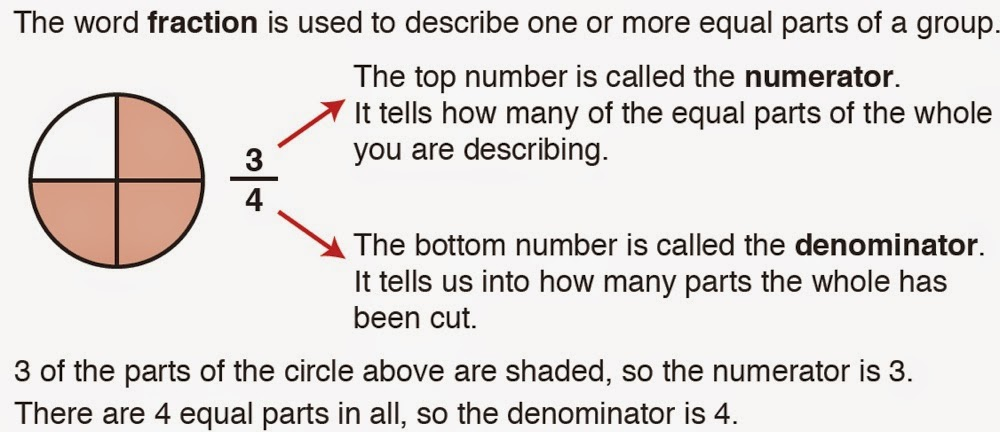## Tuesday, November 25, 2014

### Excel Math: Calming the Frenzy Over FractionsExcel Math Lesson on Fractions
Fractions have always been a nemesis for students and teachers alike.

Now with new standards, the emphasis on fractions is even greater than before.

Students are expected to master fractions sooner than ever, and Excel Math is here to help!

In Excel Math lessons, fractions are introduced in clear and concise language, starting with Grade 1 and continuing in complexity through Grade 6.

See an example of an Excel Math lesson on fractions above.

Following the brief lesson, students are given a chance to work with fractions while the teacher is nearby, ready to help those who need assistance. Fractions on a number line are now introduced to give students a visual image of how fractions are ordered.Fractions on a Number Line

Consumable pages give students lots of opportunities to practice the math problems.

Excel Math's unique CheckAnswer system lets students self assess so they can discover and correct most mistakes on their own.

During Guided Practice, students can call on a teacher to help them when they run into difficulty.After two weeks of practice, fractions are included as homework. A week or two later, fractions finally show up on the weekly test.

By this time, students are very familiar with fractions.

Repetition helps get fractions into long-term memory, which builds student confidence and success in math — including success on these new assessments.

As a result, Excel Math students are testing off the charts in mathematics.

You may also enjoy these articles:

Excel Math Helps Students Raise Test Scores

Math Placement Tests: Off to a Great Start

Five Steps to Solving Word Problems

Common Core—A New View on Teaching Math

## Monday, November 17, 2014

### Excel Math Helps Students Raise Test ScoresPrediction: Half of math students will not meet grade-level proficiency marks this spring
The Smarter Balanced Assessment Consortium today announced cut scores for its spring test, and released data projecting that more than half of students will not meet grade-level-proficiency marks in mathematics on its test this spring.

The Smarter Balanced Assessment Consortium is a group that is designing assessments for the Common Core State Standards.

Since many students are not doing well on Common Core pretests, it is easy to see why parents and teachers may be getting nervous about how well their students will do on these assessments.

Even so, Excel Math lessons continue to build student confidence and success in math— including success on these new assessments. As a result, Excel Math students are testing off the charts in mathematics.

Here's what one mom wrote to tell us:

"My children have been using Excel Math Standard Edition at home for the last year to supplement the math curriculum they have at school (which isn't very effective). This year they took the Common Core Math pretest for the first time. We had been warned that our children would probably not score very well on these tests.
However, my fourth grader scored 83% and my third grader (who is not a math genius) scored 98%!
When people asked me if he was a math whiz, I had to tell them, "Not at all. It was the Excel Math Lesson Sheets!"
— Wendy Ullrich, grateful parent

Parents across the country are discovering that Excel Math lessons help students retain concepts into long-term memory so they can recall those concepts when assessed. Other math lessons can't even compare. As a result, Excel Math students are scoring well on Iowa Basic Skills Tests, Texas STAAR tests and even Common Core pretests!